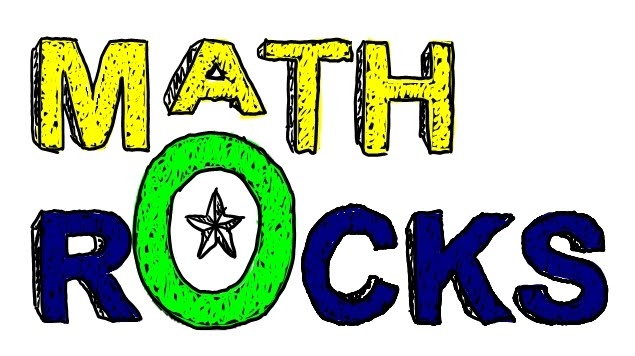•Math Fact Fluency

In second grade mastering basic math facts is a big goal that we are working towards. It is expected that by the end of second grade, students can fluently add and subtract within 20 using mental strategies. Fluency develops overtime and in three phases: counting phase, deriving phase (using reasoning strategies) and mastery.  Playing games is a great way to practice facts and helps students move through the phases.

Concepts in Math this week:

This week the children continue to work on developing their subtraction skills.

Homework

1/20- No School

1/21- mini math p 54; First In Math

1/22- mini math p 55 and 56; First In Math

1/23- mini math p 57; First In Math

1/24- First In Math